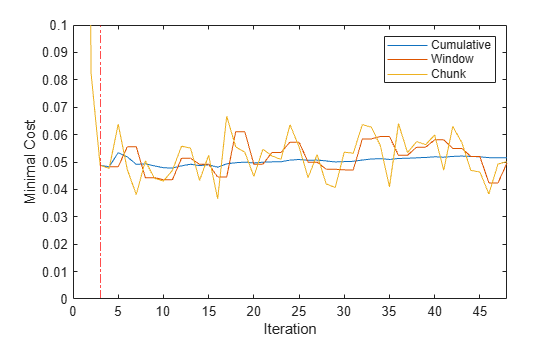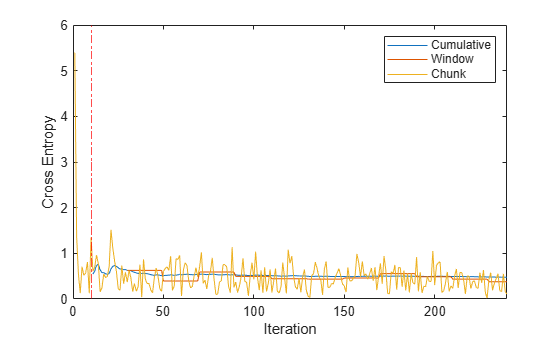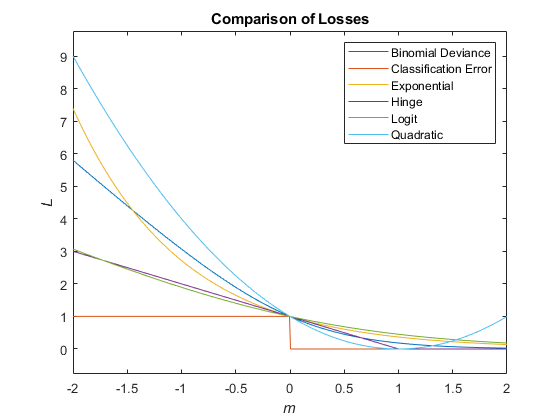loss

Loss of naive Bayes classification model for incremental learning on batch of data

Description

loss returns the classification loss of a configured naive Bayes classification model for incremental learning model (incrementalClassificationNaiveBayes object).

To measure model performance on a data stream and store the results in the output model, call updateMetrics or updateMetricsAndFit.

example

L = loss(Mdl,X,Y) returns the minimal cost classification loss for the naive Bayes classification model for incremental learning Mdl using the batch of predictor data X and corresponding responses Y.

example

L = loss(Mdl,X,Y,Name,Value) uses additional options specified by one or more name-value pair arguments. For example, you can specify the classification loss function .

Examples

collapse all

The performance of an incremental model on streaming data is measured in three ways:

1. Cumulative metrics measure the performance since the start of incremental learning.

2. Window metrics measure the performance on a specified window of observations. The metrics are updated every time the model processes the specified window.

3. The loss function measures the performance on a specified batch of data only.

Load the human activity data set. Randomly shuffle the data.

n = numel(actid);
rng(1); % For reproducibility
idx = randsample(n,n);
X = feat(idx,:);
Y = actid(idx);

For details on the data set, enter Description at the command line.

Create a naive Bayes classification model for incremental learning; specify the class names and a metrics window size of 1000 observations. Configure it for loss by fitting it to the first 10 observations.

Mdl = incrementalClassificationNaiveBayes('ClassNames',unique(Y),'MetricsWindowSize',1000);
initobs = 10;
Mdl = fit(Mdl,X(1:initobs,:),Y(1:initobs));
canComputeLoss = (size(Mdl.DistributionParameters,2) == Mdl.NumPredictors) +...
(size(Mdl.DistributionParameters,1) > 1) > 1
canComputeLoss = logical
1

Mdl is an incrementalClassificationLinear model. All its properties are read-only.

Simulate a data stream, and perform the following actions on each incoming chunk of 50 observations:

1. Call updateMetrics to measure the cumulative performance and the performance within a window of observations. Overwrite the previous incremental model with a new one to track performance metrics.

2. Call loss to measure the model performance on the incoming chunk.

3. Call fit to fit the model to the incoming chunk. Overwrite the previous incremental model with a new one fitted to the incoming observation.

4. Store all performance metrics to see how they evolve during incremental learning.

% Preallocation
numObsPerChunk = 500;
nchunk = floor((n - initobs)/numObsPerChunk);
mc = array2table(zeros(nchunk,3),'VariableNames',["Cumulative" "Window" "Loss"]);

% Incremental learning
for j = 1:nchunk
ibegin = min(n,numObsPerChunk*(j-1) + 1 + initobs);
iend   = min(n,numObsPerChunk*j + initobs);
idx = ibegin:iend;
Mdl = updateMetrics(Mdl,X(idx,:),Y(idx));
mc{j,["Cumulative" "Window"]} = Mdl.Metrics{"MinimalCost",:};
mc{j,"Loss"} = loss(Mdl,X(idx,:),Y(idx));
Mdl = fit(Mdl,X(idx,:),Y(idx));
end

Mdl is an incrementalClassificationNaiveBayes model object trained on all the data in the stream. During incremental learning and after the model is warmed up, updateMetrics checks the performance of the model on the incoming observation, then and the fit function fits the model to that observation. loss is agnostic of the metrics warm-up period, so it measures the minimal cost for all iterations.

To see how the performance metrics evolved during training, plot them.

figure;
plot(mc.Variables);
xlim([0 nchunk]);
ylim([0 0.1])
ylabel('Minimal Cost')
xline(Mdl.MetricsWarmupPeriod/numObsPerChunk + 1,'r-.');
legend(mc.Properties.VariableNames)
xlabel('Iteration')During the metrics warm-up period (the area to the left of the red line), the yellow line represents the minimal cost on each incoming chunk of data. After the metrics warm-up period, Mdl tracks the cumulative and window metrics. The cumulative and batch losses converge as the fit function fits the incremental model to the incoming data.

Fit a naive Bayes classification model for incremental learning to streaming data, and compute the multiclass cross entropy loss on the incoming chunks of data.

Load the human activity data set. Randomly shuffle the data.

n = numel(actid);
rng(1); % For reproducibility
idx = randsample(n,n);
X = feat(idx,:);
Y = actid(idx);

For details on the data set, enter Description at the command line.

Create a naive Bayes classification model for incremental learning. Configure the model as follows:

• Specify the class names

• Specify a metrics warm-up period of 1000 observations.

• Specify a metrics window size of 2000 observations.

• Track multiclass cross entropy loss to measure the performance of the model. Create an anonymous function that measures the multiclass cross entropy loss of each new observation, include a tolerance for numerical stability. Create a structure array containing the name CrossEntropy and its corresponding function.

• Configure the model to compute classification loss by fitting the model to the first 10 observations.

tolerance = 1e-10;
crossentropy = @(z,zfit,w,cost)-log(max(zfit(z),tolerance));
ce = struct("CrossEntropy",crossentropy);

Mdl = incrementalClassificationNaiveBayes('ClassNames',unique(Y),'MetricsWarmupPeriod',1000,...
'MetricsWindowSize',2000,'Metrics',ce);
initobs = 10;
Mdl = fit(Mdl,X(1:initobs,:),Y(1:initobs));

Mdl is an incrementalClassificationNaiveBayes model object configured for incremental learning.

Perform incremental learning. At each iteration:

• Simulate a data stream by processing a chunk of 100 observations.

• Call updateMetrics to compute cumulative and window metrics on the incoming chunk of data. Overwrite the previous incremental model with a new one fitted to overwrite the previous metrics.

• Call loss to compute the cross entropy on the incoming chunk of data. Whereas the cumulative and window metrics require that custom losses return the loss for each observation, loss requires the loss on the entire chunk. Compute the mean of the losses within a chunk.

• Call fit to fit the incremental model to the incoming chunk of data.

• Store the cumulative, window, and chunk metrics to see how they evolve during incremental learning.

% Preallocation
numObsPerChunk = 100;
nchunk = floor((n - initobs)/numObsPerChunk);
tanloss = array2table(zeros(nchunk,3),'VariableNames',["Cumulative" "Window" "Chunk"]);

% Incremental fitting
for j = 1:nchunk
ibegin = min(n,numObsPerChunk*(j-1) + 1 + initobs);
iend   = min(n,numObsPerChunk*j + initobs);
idx = ibegin:iend;
Mdl = updateMetrics(Mdl,X(idx,:),Y(idx));
tanloss{j,1:2} = Mdl.Metrics{"CrossEntropy",:};
tanloss{j,3} = loss(Mdl,X(idx,:),Y(idx),'LossFun',@(z,zfit,w,cost)mean(crossentropy(z,zfit,w,cost)));
Mdl = fit(Mdl,X(idx,:),Y(idx));
end

IncrementalMdl is an incrementalClassificationNaiveBayes model object trained on all the data in the stream. During incremental learning and after the model is warmed up, updateMetrics checks the performance of the model on the incoming observation, and the fit function fits the model to that observation.

Plot the performance metrics to see how they evolved during incremental learning.

figure;
h = plot(tanloss.Variables);
ylabel('Cross Entropy')
xline(Mdl.MetricsWarmupPeriod/numObsPerChunk,'r-.');
xlabel('Iteration')
legend(h,tanloss.Properties.VariableNames)The plot suggests the following:

• updateMetrics computes performance metrics after the metrics warm-up period only.

• updateMetrics computes the cumulative metrics during each iteration.

• updateMetrics computes the window metrics after processing 100 observations

• Because Mdl was configured to predict observations from the beginning of incremental learning, loss can compute the cross entropy on each incoming chunk of data.

Input Arguments

collapse all

Naive Bayes classification model for incremental learning, specified as an incrementalClassificationNaiveBayes model object. You can create Mdl directly or by converting a supported, traditionally trained machine learning model using the incrementalLearner function. For more details, see the corresponding reference page.

You must configure Mdl to compute its loss on a batch of observations.

• If Mdl is a converted, traditionally trained model, you can compute its loss without any modifications.

• Otherwise, you must fit the input model Mdl to data that contained all expected classes (Mdl.DistributionParameters must be a cell matrix with Mdl.NumPredictors columns and at least one row, where each row corresponds to each class name in Mdl.ClassNames).

Batch of predictor data with which to compute the loss, specified as an n-by-Mdl.NumPredictors floating point matrix.

The length of the observation labels Y and the number of observations in X must be equal; Y(j) is the label of observation j (row or column) in X.

Data Types: single | double

Batch of labels with which to compute the loss, specified as a categorical, character, or string array, logical or floating-point vector, or cell array of character vectors for classification problems; or a floating-point vector for regression problems.

The length of the observation labels Y and the number of observations in X must be equal; Y(j) is the label of observation j (row or column) in X.

• When the ClassNames property of the input model Mdl is nonempty, the following conditions apply:

• If Y contains a label that is not a member of Mdl.ClassNames, loss issues an error.

• The data type of Y and Mdl.ClassNames must be the same.

Data Types: char | string | cell | categorical | logical | single | double

Name-Value Arguments

Specify optional comma-separated pairs of Name,Value arguments. Name is the argument name and Value is the corresponding value. Name must appear inside quotes. You can specify several name and value pair arguments in any order as Name1,Value1,...,NameN,ValueN.

Example: 'LossFun','classiferror','Weights',W specifies returning the misclassification error rate, and the observation weights W.

Cost of misclassifying an observation, specified as a value in the table, where c is the number of classes in Mdl.ClassNames. The specified value overrides the value of Mdl.Cost.

ValueDescription
c-by-c numeric matrix

Cost(i,j) is the cost of classifying an observation into class j when its true class is i, for classes Mdl.ClassNames(i) and Mdl.ClassNames(j). In other words, the rows correspond to the true class and the columns correspond to the predicted class. For example, Cost = [0 2;1 0] applies double the penalty for misclassifying Mdl.ClassNames(1) than for misclassifying Mdl.ClassNames(2).

Structure array

A structure array having two fields:

• ClassNames containing the class names, the same value as Mdl.ClassNames

• ClassificationCosts containing the cost matrix, as previously described.

Example: Cost=struct('ClassNames',Mdl.ClassNames,'ClassificationCosts',[0 2; 1 0])

Data Types: single | double | struct

Loss function, specified as a built-in loss function name or function handle.

The following table lists the built-in loss function names. You can specify more than one by using a string vector.

NameDescription
"binodeviance"Binomial deviance
"classiferror"Misclassification error rate
"exponential"Exponential
"hinge"Hinge
"logit"Logistic
'"mincost"

Minimal expected misclassification cost

For more details, see Classification Loss.

To specify a custom loss function, use function handle notation. The function must have this form:

lossval = lossfcn(C,S,W,Cost)

• The output argument lossval is an n-by-1 floating-point vector, where lossval(j) is the classification loss of observation j.

• You specify the function name (lossfcn).

• C is an n-by-2 logical matrix with rows indicating the class to which the corresponding observation belongs. The column order corresponds to the class order in the ClassNames property. Create C by setting C(p,q) = 1, if observation p is in class q, for each observation in the specified data. Set the other element in row p to 0.

• S is an n-by-2 numeric matrix of predicted classification scores. S is similar to the Posterior output of predict, where rows correspond to observations in the data and the column order corresponds to the class order in the ClassNames property. S(p,q) is the classification score of observation p being classified in class q.

• W is an n-by-1 numeric vector of observation weights.

• Cost is a K-by-K numeric matrix of misclassification costs.

Example: 'LossFun',"classiferror"

Example: 'LossFun',@lossfcn

Data Types: char | string | function_handle

Prior class probabilities, specified as a value in this numeric vector. Prior has the same length as the number of classes in Mdl.ClassNames, and the order of the elements corresponds to the class order in Mdl.ClassNames. loss normalizes the vector so that the sum of the result is 1.

The specified value overrides the value of Mdl.Prior.

Data Types: single | double

Score transformation function describing how incremental learning functions transform raw response values, specified as a character vector, string scalar, or function handle. The specified value overrides the value of Mdl.ScoreTransform.

This table describes the available built-in functions for score transformation.

ValueDescription
"doublelogit"1/(1 + e–2x)
"invlogit"log(x / (1 – x))
"ismax"Sets the score for the class with the largest score to 1, and sets the scores for all other classes to 0
"logit"1/(1 + ex)
"none" or "identity"x (no transformation)
"sign"–1 for x < 0
0 for x = 0
1 for x > 0
"symmetric"2x – 1
"symmetricismax"Sets the score for the class with the largest score to 1, and sets the scores for all other classes to –1
"symmetriclogit"2/(1 + ex) – 1

Data Types: char | string

Chunk of observation weights, specified as a floating-point vector of positive values. loss weighs the observations in X with the corresponding values in Weights. The size of Weights must equal n, which is the number of observations in X.

By default, Weights is ones(n,1).

For more details, including normalization schemes, see Observation Weights.

Data Types: double | single

Output Arguments

collapse all

Classification loss, returned as a numeric scalar. L is a measure of model quality. Its interpretation depends on the loss function and weighting scheme.

collapse all

Classification Loss

Classification loss functions measure the predictive inaccuracy of classification models. When you compare the same type of loss among many models, a lower loss indicates a better predictive model.

Consider the following scenario.

• L is the weighted average classification loss.

• n is the sample size.

• For binary classification:

• yj is the observed class label. The software codes it as –1 or 1, indicating the negative or positive class (or the first or second class in the ClassNames property), respectively.

• f(Xj) is the positive-class classification score for observation (row) j of the predictor data X.

• mj = yjf(Xj) is the classification score for classifying observation j into the class corresponding to yj. Positive values of mj indicate correct classification and do not contribute much to the average loss. Negative values of mj indicate incorrect classification and contribute significantly to the average loss.

• For algorithms that support multiclass classification (that is, K ≥ 3):

• yj* is a vector of K – 1 zeros, with 1 in the position corresponding to the true, observed class yj. For example, if the true class of the second observation is the third class and K = 4, then y2* = [0 0 1 0]′. The order of the classes corresponds to the order in the ClassNames property of the input model.

• f(Xj) is the length K vector of class scores for observation j of the predictor data X. The order of the scores corresponds to the order of the classes in the ClassNames property of the input model.

• mj = yj*f(Xj). Therefore, mj is the scalar classification score that the model predicts for the true, observed class.

• The weight for observation j is wj. The software normalizes the observation weights so that they sum to the corresponding prior class probability. The software also normalizes the prior probabilities so they sum to 1. Therefore,

$\sum _{j=1}^{n}{w}_{j}=1.$

Given this scenario, the following table describes the supported loss functions that you can specify by using the 'LossFun' name-value pair argument.

Loss FunctionValue of LossFunEquation
Binomial deviance'binodeviance'$L=\sum _{j=1}^{n}{w}_{j}\mathrm{log}\left\{1+\mathrm{exp}\left[-2{m}_{j}\right]\right\}.$
Misclassified rate in decimal'classiferror'

$L=\sum _{j=1}^{n}{w}_{j}I\left\{{\stackrel{^}{y}}_{j}\ne {y}_{j}\right\}.$

${\stackrel{^}{y}}_{j}$ is the class label corresponding to the class with the maximal score. I{·} is the indicator function.

Cross-entropy loss'crossentropy'

'crossentropy' is appropriate only for neural network models.

The weighted cross-entropy loss is

$L=-\sum _{j=1}^{n}\frac{{\stackrel{˜}{w}}_{j}\mathrm{log}\left({m}_{j}\right)}{Kn},$

where the weights ${\stackrel{˜}{w}}_{j}$ are normalized to sum to n instead of 1.

Exponential loss'exponential'$L=\sum _{j=1}^{n}{w}_{j}\mathrm{exp}\left(-{m}_{j}\right).$
Hinge loss'hinge'$L=\sum _{j=1}^{n}{w}_{j}\mathrm{max}\left\{0,1-{m}_{j}\right\}.$
Logit loss'logit'$L=\sum _{j=1}^{n}{w}_{j}\mathrm{log}\left(1+\mathrm{exp}\left(-{m}_{j}\right)\right).$
Minimal expected misclassification cost'mincost'

'mincost' is appropriate only if classification scores are posterior probabilities.

The software computes the weighted minimal expected classification cost using this procedure for observations j = 1,...,n.

1. Estimate the expected misclassification cost of classifying the observation Xj into the class k:

${\gamma }_{jk}={\left(f{\left({X}_{j}\right)}^{\prime }C\right)}_{k}.$

f(Xj) is the column vector of class posterior probabilities for binary and multiclass classification for the observation Xj. C is the cost matrix stored in the Cost property of the model.

2. For observation j, predict the class label corresponding to the minimal expected misclassification cost:

${\stackrel{^}{y}}_{j}=\underset{k=1,...,K}{\text{argmin}}{\gamma }_{jk}.$

3. Using C, identify the cost incurred (cj) for making the prediction.

The weighted average of the minimal expected misclassification cost loss is

$L=\sum _{j=1}^{n}{w}_{j}{c}_{j}.$

If you use the default cost matrix (whose element value is 0 for correct classification and 1 for incorrect classification), then the 'mincost' loss is equivalent to the 'classiferror' loss.

Quadratic loss'quadratic'$L=\sum _{j=1}^{n}{w}_{j}{\left(1-{m}_{j}\right)}^{2}.$

This figure compares the loss functions (except 'crossentropy' and 'mincost') over the score m for one observation. Some functions are normalized to pass through the point (0,1).Algorithms

collapse all

Observation Weights

For each conditional predictor distribution, loss computes the weighted average and standard deviation.

If the prior class probability distribution is known (in other words, the prior distribution is not empirical), loss normalizes observation weights to sum to the prior class probabilities in the respective classes. This action implies that the default observation weights are the respective prior class probabilities.

If the prior class probability distribution is empirical, the software normalizes the specified observation weights to sum to 1 each time you call loss.